# Kaplansky field

A fieldequipped with a valuationand satisfying Kaplansky's hypothesis A, as introduced in [a2]. Letdenote the characteristic of the residue field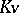if it is a positive prime; otherwise, set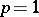. Hypothesis A requires that:

i) the value group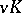is a-divisible group;

ii) for every additive polynomial (cf. [a5])with coefficients inand every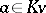, the equationhas a solution in. Requirement ii) implies thatis a perfect field. Using Galois cohomology, G. Whaples [a6] showed that ii) means that the degree of every finite extension ofis prime to. An elementary proof of this was given by F. Delon in 1981.

I. Kaplansky considered immediate extensions (cf. Valuation) of fields with valuations. He used pseudo-Cauchy sequences (also called Ostrowski nets), which were introduced by A. Ostrowski in 1935. These are analogues of Cauchy sequences (cf. Cauchy sequence) for the metric induced by the valuation, but their limits need not be unique; for this reason, they are called pseudo-limits.

In [a3], W. Krull proved that there always exist maximal immediate extensions (in order to apply the Zorn lemma, Krull gave an upper bound for the cardinality of immediate extensions of a fixed field; an elegant deduction of this bound was later given by K.A.H. Gravett in [a1]). Kaplansky showed that if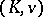satisfies hypothesis A, then its maximal immediate extensions are unique up to a valuation-preserving isomorphism over. The same holds for the maximal immediate algebraic extensions, and this fact can also be shown via a Galois-theoretic interpretation of hypothesis A (cf. [a4]). The former result follows from the latter by a theorem of Kaplansky (which has a certain analogue in the theory of real closed fields, cf. Real closed field): Ifhas no non-trivial immediate algebraic extensions, then the isomorphism type of an immediate extensionoveris determined by the pseudo-Cauchy sequences inthat have pseudo-limit.